# The average

The average of one set of 4 numbers is 35. The average of another set of number is 20. The average of the numbers in the two sets is 30. How many numbers are there in the other set?

n =  2

### Step-by-step explanation: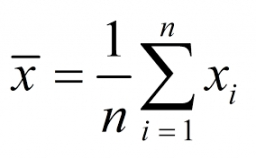Did you find an error or inaccuracy? Feel free to write us. Thank you!Tips to related online calculators
Looking for help with calculating arithmetic mean?
Looking for a statistical calculator?
Do you have a linear equation or system of equations and looking for its solution? Or do you have a quadratic equation?

#### You need to know the following knowledge to solve this word math problem:

We encourage you to watch this tutorial video on this math problem:

## Related math problems and questions:

• Arithmetic averageThe arithmetic mean of the five numbers is exactly 8. The sum of these four numbers is 30. What is the fifth number?
• The rawThe raw data presented here are the scores (out of 100 marks) of a market survey regarding the acceptability of new product launched by a company for random sample of 50 respondents: 40 45 41 45 45 30 30 8 48 25 26 9 23 24 26 29 8 40 41 42 39 35 18 25 35
• ComplaintsThe table is given: days complaints 0-4 2 5-9 4 10-14 8 15-19 6 20-24 4 25-29 3 30-34 3 1.1 What percentage of complaints were resolved within 2weeks? 1.2 calculate the mean number of days to resolve these complaints. 1.3 calculate the modal number of day
• Brothers and sisters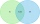There are 35 children in the class, 23 of them have a brother, and 27 of them have a sister. How many children have both a brother and a sister when there are 5 children in the class who have no brother or sister?
• Dance ensembles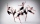4 dance ensembles were dancing at the festival. None had less than 10 and more than 20 members. All dancers from some of the two ensembles were represented in each dance. First, 31 participants were on the stage, then 32, 34, 35, 37, and 38. How many danc
• Lunch timeIn a cafeteria, 3/10 of the students are eating salads, and 3/5 are eating sandwiches. There are 30 students in the cafeteria. How many students are eating lunches other than salads or sandwiches?
• Classroom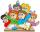In a class are 32 pupils. Boys are 8 less than girls. How many boys and girls are in the classroom?
• RemaindersIt is given a set of numbers { 170; 244; 299; 333; 351; 391; 423; 644 }. Divide this numbers by number 66 and determine set of remainders. As result write sum of this remainders.
• TouristTourist walk at 4.1 km/h. Every 5 km have 20-minute break. How many kilometers passed, when started at 4:40 and finished at 10:30?
• NumberCalculate the integer number which, divided by 34 gives 10 and the rest 25.
• Divisible by 5How many three-digit odd numbers divisible by 5, which are in place ten's number 3?
• Pets in ZOOThe zoo have run for pets. They are chickens, pigs and cow. Altogether there has 20 heads and 60 feet. How many chickens and piglets are there?
• Subtracting sets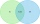For two sets K, L is true: K has 30 elements, L has 27 elements and the set L - K has 22 elements. How many elements does the set K - L have?
• Here isHere is a data set (n=117) that has been sorted. 10.4 12.2 14.3 15.3 17.1 17.8 18 18.6 19.1 19.9 19.9 20.3 20.6 20.7 20.7 21.2 21.3 22 22.1 22.3 22.8 23 23 23.1 23.5 24.1 24.1 24.4 24.5 24.8 24.9 25.4 25.4 25.5 25.7 25.9 26 26.1 26.2 26.7 26.8 27.5 27.6 2
• Fractions and mixed numerals(a) Convert the following mixed numbers to improper fractions. i. 3 5/8 ii. 7 7/6 (b) Convert the following improper fraction to mixed number. i. 13/4 ii. 78/5 (c) Simplify these fractions to their lowest terms. i. 36/42 ii. 27/45 2. evaluate following ex
• Dinosaurs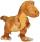More than 30 and less than 60 dinosaurs have met at the pond. A quarter of them bathed and 1/7 saws, and the rest gripped. How many were at the pond? How many were there?
• Birthdate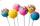Jane on birthday brought 30 lollipops and 24 chewing gum for their friends. How many friends has, if everyone receives the same number of lollipops and chewing gums? How much chewing gum and lollipops got any friend?